# Determination of shock losses and pressure losses in ug mine openings (1)

29 Dec 2019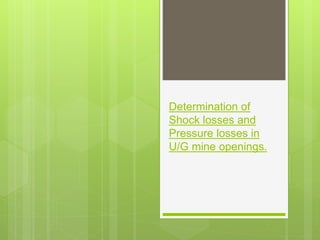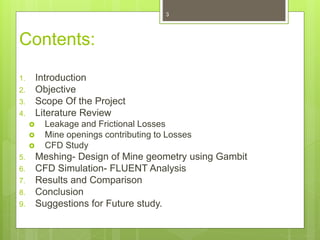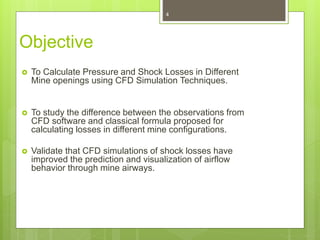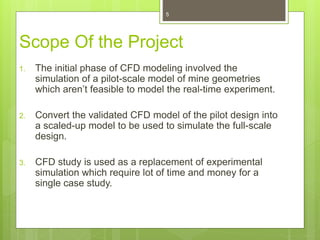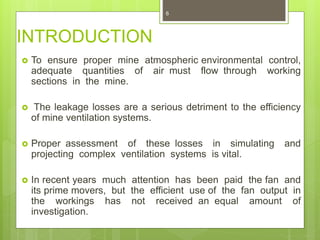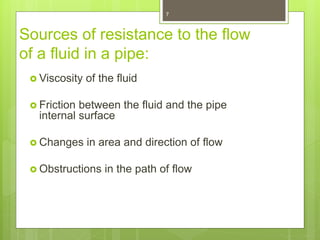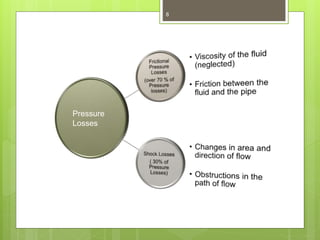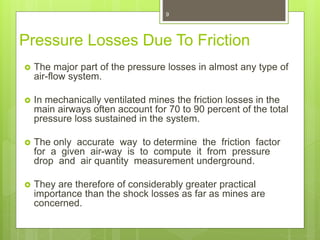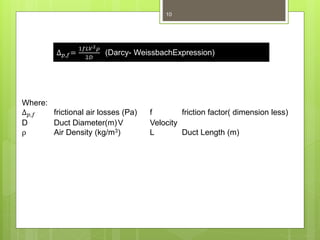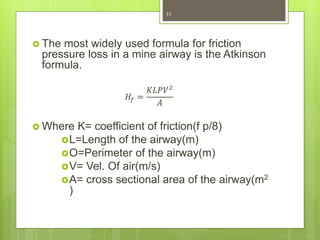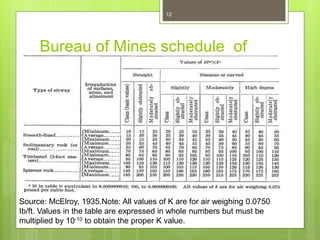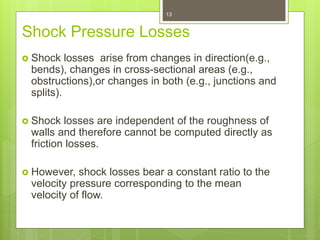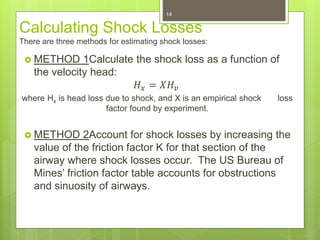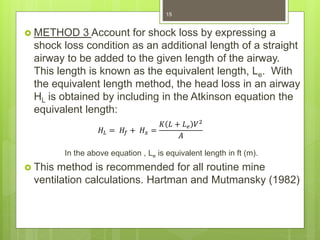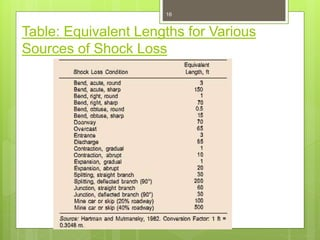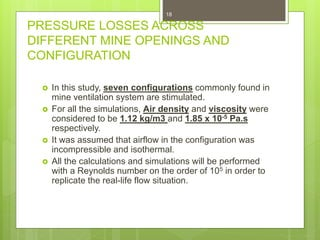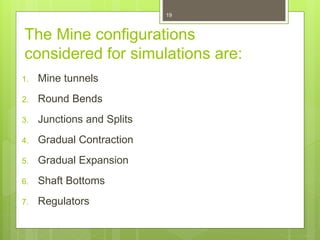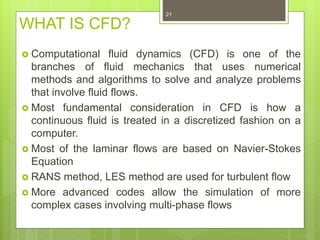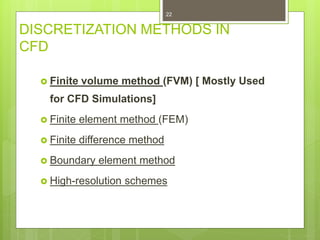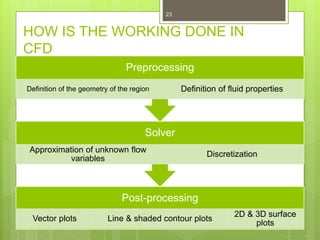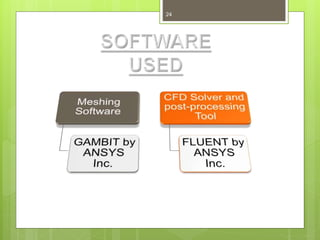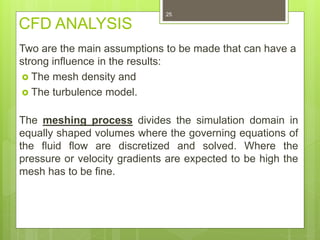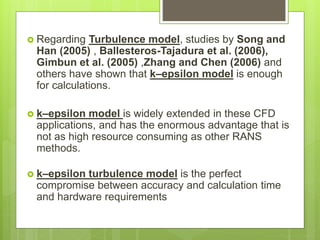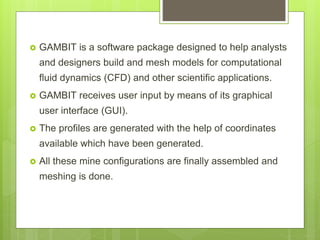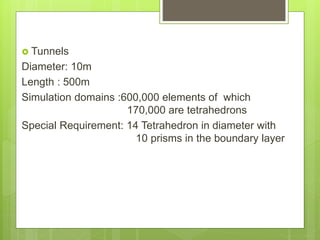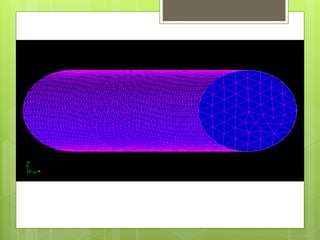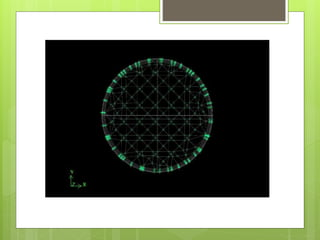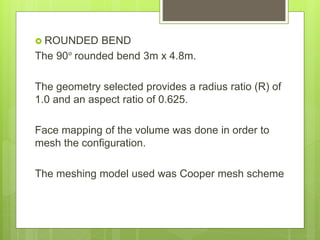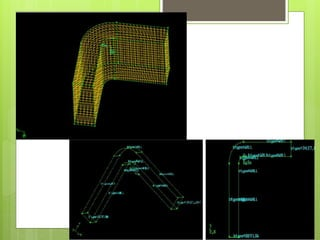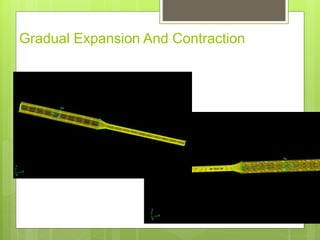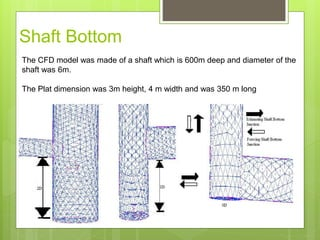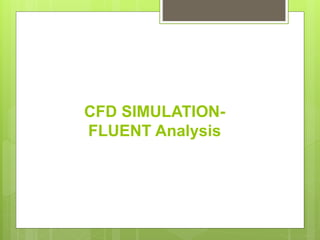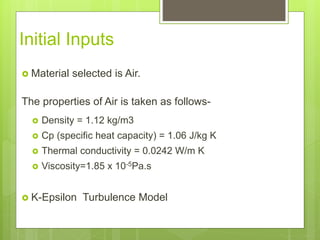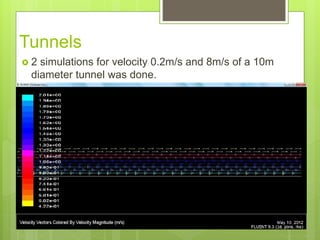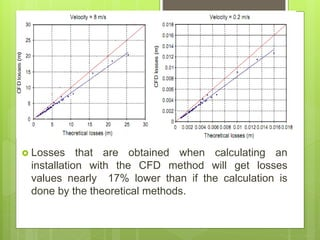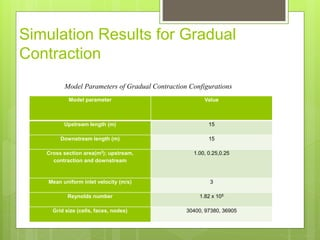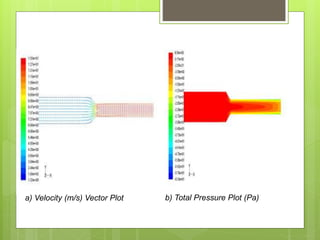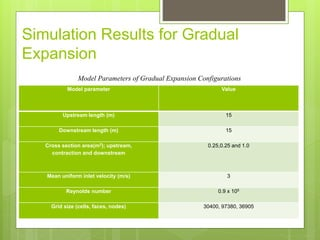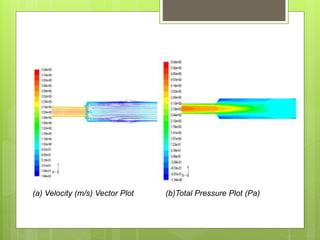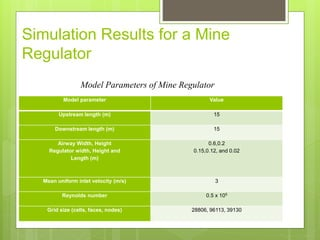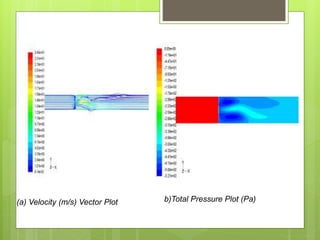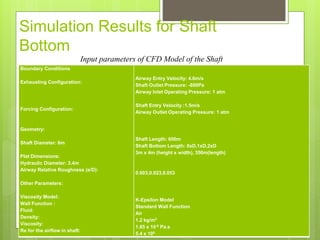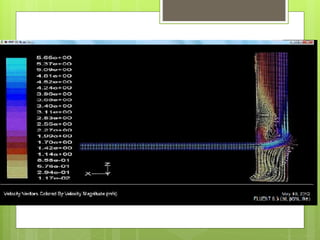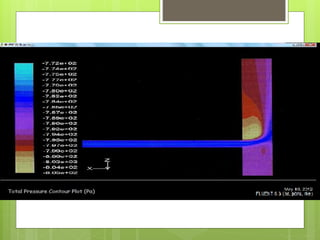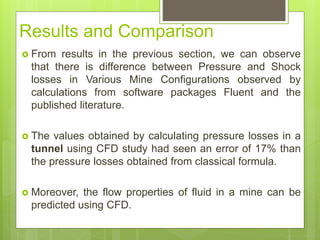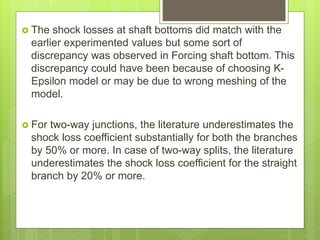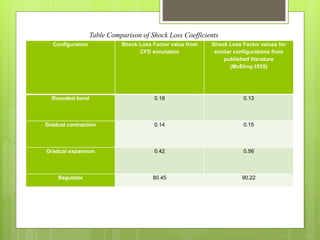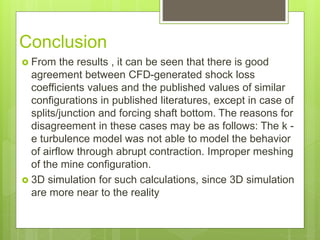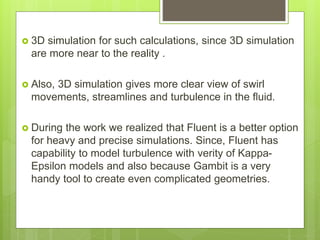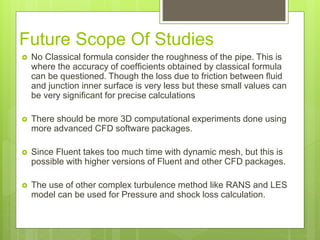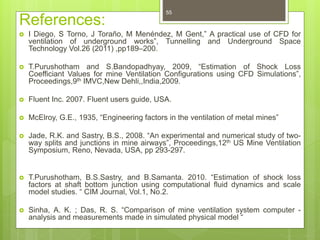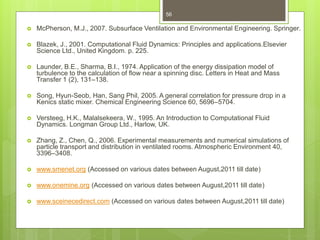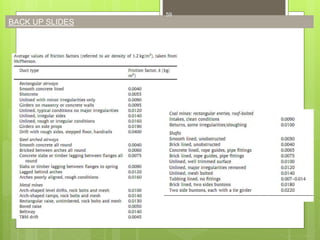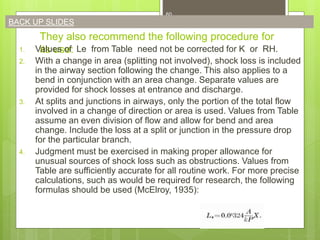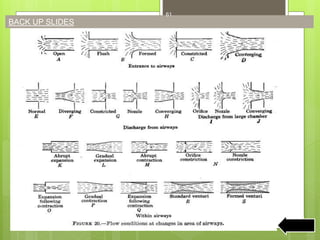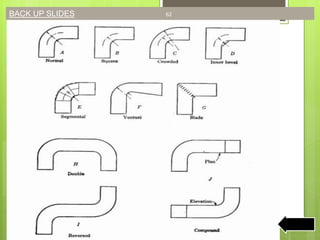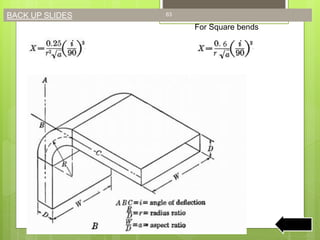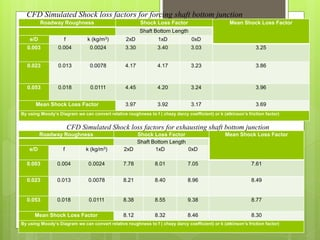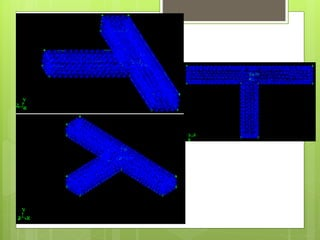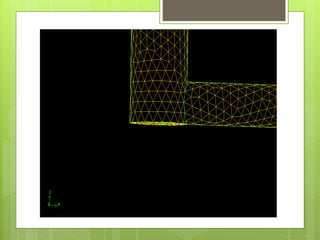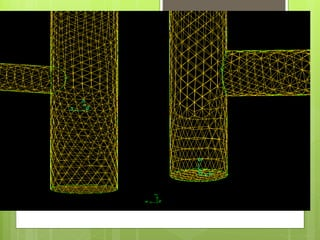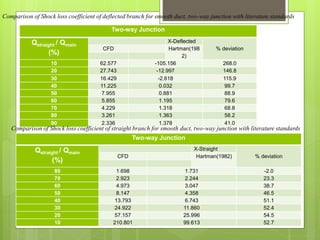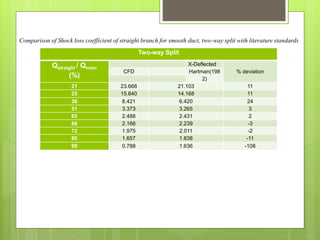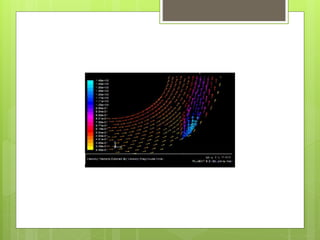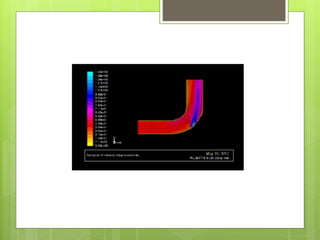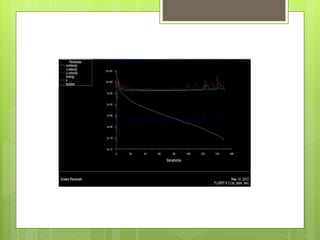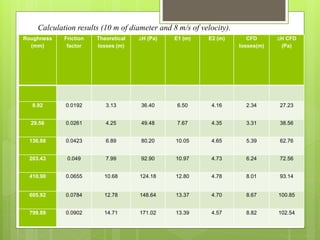1 sur 74

### Determination of shock losses and pressure losses in ug mine openings (1)

• 2. Determination of Shock losses and Pressure losses in U/G mine openings.
• 3. Contents: 1. Introduction 2. Objective 3. Scope Of the Project 4. Literature Review  Leakage and Frictional Losses  Mine openings contributing to Losses  CFD Study 5. Meshing- Design of Mine geometry using Gambit 6. CFD Simulation- FLUENT Analysis 7. Results and Comparison 8. Conclusion 9. Suggestions for Future study. 3
• 4. Objective  To Calculate Pressure and Shock Losses in Different Mine openings using CFD Simulation Techniques.  To study the difference between the observations from CFD software and classical formula proposed for calculating losses in different mine configurations.  Validate that CFD simulations of shock losses have improved the prediction and visualization of airflow behavior through mine airways. 4
• 5. Scope Of the Project 1. The initial phase of CFD modeling involved the simulation of a pilot-scale model of mine geometries which aren’t feasible to model the real-time experiment. 2. Convert the validated CFD model of the pilot design into a scaled-up model to be used to simulate the full-scale design. 3. CFD study is used as a replacement of experimental simulation which require lot of time and money for a single case study. 5
• 6. INTRODUCTION  To ensure proper mine atmospheric environmental control, adequate quantities of air must flow through working sections in the mine.  The leakage losses are a serious detriment to the efficiency of mine ventilation systems.  Proper assessment of these losses in simulating and projecting complex ventilation systems is vital.  In recent years much attention has been paid the fan and its prime movers, but the efficient use of the fan output in the workings has not received an equal amount of investigation. 6
• 7. Sources of resistance to the flow of a fluid in a pipe:  Viscosity of the fluid  Friction between the fluid and the pipe internal surface  Changes in area and direction of flow  Obstructions in the path of flow 7
• 8. Pressure Losses 8
• 9. Pressure Losses Due To Friction  The major part of the pressure losses in almost any type of air-flow system.  In mechanically ventilated mines the friction losses in the main airways often account for 70 to 90 percent of the total pressure loss sustained in the system.  The only accurate way to determine the friction factor for a given air-way is to compute it from pressure drop and air quantity measurement underground.  They are therefore of considerably greater practical importance than the shock losses as far as mines are concerned. 9
• 10. Where: ∆ 𝑝,𝑓 frictional air losses (Pa) f friction factor( dimension less) D Duct Diameter(m)V Velocity ρ Air Density (kg/m3) L Duct Length (m) 10 ∆ 𝑝,𝑓= 1𝑓𝐿𝑉2 𝜌 2𝐷 (Darcy- WeissbachExpression))
• 11.  The most widely used formula for friction pressure loss in a mine airway is the Atkinson formula. 𝐻𝑓 = 𝐾𝐿𝑃𝑉2 𝐴  Where K= coefficient of friction(f p/8) L=Length of the airway(m) O=Perimeter of the airway(m) V= Vel. Of air(m/s) A= cross sectional area of the airway(m2 ) 11
• 12. Bureau of Mines schedule of friction factors for mine airways 12 Source: McElroy, 1935.Note: All values of K are for air weighing 0.0750 Ib/ft. Values in the table are expressed in whole numbers but must be multiplied by 10-10 to obtain the proper K value.
• 13. Shock Pressure Losses  Shock losses arise from changes in direction(e.g., bends), changes in cross-sectional areas (e.g., obstructions),or changes in both (e.g., junctions and splits).  Shock losses are independent of the roughness of walls and therefore cannot be computed directly as friction losses.  However, shock losses bear a constant ratio to the velocity pressure corresponding to the mean velocity of flow. 13
• 14. Calculating Shock Losses There are three methods for estimating shock losses:  METHOD 1Calculate the shock loss as a function of the velocity head: 𝐻 𝑥 = 𝑋𝐻𝑣 where Hx is head loss due to shock, and X is an empirical shock loss factor found by experiment.  METHOD 2Account for shock losses by increasing the value of the friction factor K for that section of the airway where shock losses occur. The US Bureau of Mines’ friction factor table accounts for obstructions and sinuosity of airways. 14
• 15.  METHOD 3 Account for shock loss by expressing a shock loss condition as an additional length of a straight airway to be added to the given length of the airway. This length is known as the equivalent length, Le. With the equivalent length method, the head loss in an airway HL is obtained by including in the Atkinson equation the equivalent length: In the above equation , Le is equivalent length in ft (m).  This method is recommended for all routine mine ventilation calculations. Hartman and Mutmansky (1982) 15 𝐻𝐿 = 𝐻𝑓 + 𝐻 𝑥 = 𝐾 𝐿 + 𝐿 𝑒 𝑉2 𝐴
• 16. Table: Equivalent Lengths for Various Sources of Shock Loss 16
• 17. Types of Mine Openings and Configurations Contributing to Losses in an Underground Mine 17
• 18. PRESSURE LOSSES ACROSS DIFFERENT MINE OPENINGS AND CONFIGURATION  In this study, seven configurations commonly found in mine ventilation system are stimulated.  For all the simulations, Air density and viscosity were considered to be 1.12 kg/m3 and 1.85 x 10-5 Pa.s respectively.  It was assumed that airflow in the configuration was incompressible and isothermal.  All the calculations and simulations will be performed with a Reynolds number on the order of 105 in order to replicate the real-life flow situation. 18
• 19. The Mine configurations considered for simulations are: 1. Mine tunnels 2. Round Bends 3. Junctions and Splits 4. Gradual Contraction 5. Gradual Expansion 6. Shaft Bottoms 7. Regulators 19
• 20. CFD STUDY 20
• 21. WHAT IS CFD?  Computational fluid dynamics (CFD) is one of the branches of fluid mechanics that uses numerical methods and algorithms to solve and analyze problems that involve fluid flows.  Most fundamental consideration in CFD is how a continuous fluid is treated in a discretized fashion on a computer.  Most of the laminar flows are based on Navier-Stokes Equation  RANS method, LES method are used for turbulent flow  More advanced codes allow the simulation of more complex cases involving multi-phase flows 21
• 22. DISCRETIZATION METHODS IN CFD  Finite volume method (FVM) [ Mostly Used for CFD Simulations]  Finite element method (FEM)  Finite difference method  Boundary element method  High-resolution schemes 22
• 23. HOW IS THE WORKING DONE IN CFD Post-processing Vector plots Line & shaded contour plots 2D & 3D surface plots Solver Approximation of unknown flow variables Discretization Preprocessing Definition of the geometry of the region Definition of fluid properties 23
• 24. 24
• 25. CFD ANALYSIS Two are the main assumptions to be made that can have a strong inﬂuence in the results:  The mesh density and  The turbulence model. The meshing process divides the simulation domain in equally shaped volumes where the governing equations of the ﬂuid ﬂow are discretized and solved. Where the pressure or velocity gradients are expected to be high the mesh has to be ﬁne. 25
• 26.  Regarding Turbulence model, studies by Song and Han (2005) , Ballesteros-Tajadura et al. (2006), Gimbun et al. (2005) ,Zhang and Chen (2006) and others have shown that k–epsilon model is enough for calculations.  k–epsilon model is widely extended in these CFD applications, and has the enormous advantage that is not as high resource consuming as other RANS methods.  k–epsilon turbulence model is the perfect compromise between accuracy and calculation time and hardware requirements
• 27. MESHING – Design of Configurations using GAMBIT 27
• 28.  GAMBIT is a software package designed to help analysts and designers build and mesh models for computational fluid dynamics (CFD) and other scientific applications.  GAMBIT receives user input by means of its graphical user interface (GUI).  The profiles are generated with the help of coordinates available which have been generated.  All these mine configurations are finally assembled and meshing is done.
• 29.  Tunnels Diameter: 10m Length : 500m Simulation domains :600,000 elements of which 170,000 are tetrahedrons Special Requirement: 14 Tetrahedron in diameter with 10 prisms in the boundary layer
• 32.  ROUNDED BEND The 90° rounded bend 3m x 4.8m. The geometry selected provides a radius ratio (R) of 1.0 and an aspect ratio of 0.625. Face mapping of the volume was done in order to mesh the configuration. The meshing model used was Cooper mesh scheme
• 34. Gradual Expansion And Contraction
• 35. Shaft Bottom The CFD model was made of a shaft which is 600m deep and diameter of the shaft was 6m. The Plat dimension was 3m height, 4 m width and was 350 m long
• 36. CFD SIMULATION- FLUENT Analysis
• 37. Initial Inputs  Material selected is Air. The properties of Air is taken as follows-  Density = 1.12 kg/m3  Cp (specific heat capacity) = 1.06 J/kg K  Thermal conductivity = 0.0242 W/m K  Viscosity=1.85 x 10-5Pa.s  K-Epsilon Turbulence Model
• 38. Tunnels  2 simulations for velocity 0.2m/s and 8m/s of a 10m diameter tunnel was done.
• 39.  Losses that are obtained when calculating an installation with the CFD method will get losses values nearly 17% lower than if the calculation is done by the theoretical methods.
• 40. Simulation Results for Gradual Contraction Model parameter Value Upstream length (m) 15 Downstream length (m) 15 Cross section area(m2); upstream, contraction and downstream 1.00, 0.25,0.25 Mean uniform inlet velocity (m/s) 3 Reynolds number 1.82 x 105 Grid size (cells, faces, nodes) 30400, 97380, 36905 Model Parameters of Gradual Contraction Configurations
• 41. a) Velocity (m/s) Vector Plot b) Total Pressure Plot (Pa)
• 42. Simulation Results for Gradual Expansion Model parameter Value Upstream length (m) 15 Downstream length (m) 15 Cross section area(m2); upstream, contraction and downstream 0.25,0.25 and 1.0 Mean uniform inlet velocity (m/s) 3 Reynolds number 0.9 x 105 Grid size (cells, faces, nodes) 30400, 97380, 36905 Model Parameters of Gradual Expansion Configurations
• 43. (a) Velocity (m/s) Vector Plot (b)Total Pressure Plot (Pa)
• 44. Simulation Results for a Mine Regulator Model parameter Value Upstream length (m) 15 Downstream length (m) 15 Airway Width, Height Regulator width, Height and Length (m) 0.6,0.2 0.15,0.12, and 0.02 Mean uniform inlet velocity (m/s) 3 Reynolds number 0.5 x 105 Grid size (cells, faces, nodes) 28806, 96113, 39130 Model Parameters of Mine Regulator
• 45. (a) Velocity (m/s) Vector Plot b)Total Pressure Plot (Pa)
• 46. Simulation Results for Shaft Bottom Boundary Conditions Exhausting Configuration: Forcing Configuration: Geometry: Shaft Diameter: 6m Plat Dimensions: Hydraulic Diameter: 3.4m Airway Relative Roughness (e/D): Other Parameters: Viscosity Model: Wall Function : Fluid: Density: Viscosity: Re for the airflow in shaft: Airway Entry Velocity: 4.6m/s Shaft Outlet Pressure: -800Pa Airway Inlet Operating Pressure: 1 atm Shaft Entry Velocity :1.5m/s Airway Outlet Operating Pressure: 1 atm Shaft Length: 600m Shaft Bottom Length: 0xD,1xD,2xD 3m x 4m (height x width), 350m(length) 0.003,0.023,0.053 K-Epsilon Model Standard Wall Function Air 1.2 kg/m3 1.85 x 10-5 Pa.s 5.4 x 105 Input parameters of CFD Model of the Shaft
• 49. Results and Comparison  From results in the previous section, we can observe that there is difference between Pressure and Shock losses in Various Mine Configurations observed by calculations from software packages Fluent and the published literature.  The values obtained by calculating pressure losses in a tunnel using CFD study had seen an error of 17% than the pressure losses obtained from classical formula.  Moreover, the flow properties of fluid in a mine can be predicted using CFD.
• 50.  The shock losses at shaft bottoms did match with the earlier experimented values but some sort of discrepancy was observed in Forcing shaft bottom. This discrepancy could have been because of choosing K- Epsilon model or may be due to wrong meshing of the model.  For two-way junctions, the literature underestimates the shock loss coefficient substantially for both the branches by 50% or more. In case of two-way splits, the literature underestimates the shock loss coefficient for the straight branch by 20% or more.
• 51. Configuration Shock Loss Factor value from CFD simulation Shock Loss Factor values for similar configurations from published literature (McElroy,1935) Rounded bend 0.18 0.13 Gradual contraction 0.14 0.15 Gradual expansion 0.42 0.56 Regulator 80.45 90.22 Table Comparison of Shock Loss Coefficients
• 52. Conclusion  From the results , it can be seen that there is good agreement between CFD-generated shock loss coefficients values and the published values of similar configurations in published literatures, except in case of splits/junction and forcing shaft bottom. The reasons for disagreement in these cases may be as follows: The k - e turbulence model was not able to model the behavior of airflow through abrupt contraction. Improper meshing of the mine configuration.  3D simulation for such calculations, since 3D simulation are more near to the reality
• 53.  3D simulation for such calculations, since 3D simulation are more near to the reality .  Also, 3D simulation gives more clear view of swirl movements, streamlines and turbulence in the ﬂuid.  During the work we realized that Fluent is a better option for heavy and precise simulations. Since, Fluent has capability to model turbulence with verity of Kappa- Epsilon models and also because Gambit is a very handy tool to create even complicated geometries.
• 54. Future Scope Of Studies  No Classical formula consider the roughness of the pipe. This is where the accuracy of coefﬁcients obtained by classical formula can be questioned. Though the loss due to friction between ﬂuid and junction inner surface is very less but these small values can be very signiﬁcant for precise calculations  There should be more 3D computational experiments done using more advanced CFD software packages.  Since Fluent takes too much time with dynamic mesh, but this is possible with higher versions of Fluent and other CFD packages.  The use of other complex turbulence method like RANS and LES model can be used for Pressure and shock loss calculation.
• 55. References:  I Diego, S Torno, J Toraño, M Menéndez, M Gent,” A practical use of CFD for ventilation of underground works”, Tunnelling and Underground Space Technology Vol.26 (2011) ,pp189–200.  T.Purushotham and S.Bandopadhyay, 2009, “Estimation of Shock Loss Coefficiant Values for mine Ventilation Configurations using CFD Simulations”, Proceedings,9th IMVC,New Dehli,,India,2009.  Fluent Inc. 2007. Fluent users guide, USA.  McElroy, G.E., 1935, “Engineering factors in the ventilation of metal mines”  Jade, R.K. and Sastry, B.S., 2008. “An experimental and numerical study of two- way splits and junctions in mine airways”, Proceedings,12th US Mine Ventilation Symposium, Reno, Nevada, USA, pp 293-297.  T.Purushotham, B.S.Sastry, and B.Samanta. 2010. “Estimation of shock loss factors at shaft bottom junction using computational fluid dynamics and scale model studies. “ CIM Journal, Vol.1, No.2.  Sinha, A. K. ; Das, R. S. “Comparison of mine ventilation system computer - analysis and measurements made in simulated physical model “ 55
• 56.  McPherson, M.J., 2007. Subsurface Ventilation and Environmental Engineering. Springer.  Blazek, J., 2001. Computational Fluid Dynamics: Principles and applications.Elsevier Science Ltd., United Kingdom. p. 225.  Launder, B.E., Sharma, B.I., 1974. Application of the energy dissipation model of turbulence to the calculation of ﬂow near a spinning disc. Letters in Heat and Mass Transfer 1 (2), 131–138.  Song, Hyun-Seob, Han, Sang Phil, 2005. A general correlation for pressure drop in a Kenics static mixer. Chemical Engineering Science 60, 5696–5704.  Versteeg, H.K., Malalsekeera, W., 1995. An Introduction to Computational Fluid Dynamics. Longman Group Ltd., Harlow, UK.  Zhang, Z., Chen, Q., 2006. Experimental measurements and numerical simulations of particle transport and distribution in ventilated rooms. Atmospheric Environment 40, 3396–3408.  www.smenet.org (Accessed on various dates between August,2011 till date)  www.onemine.org (Accessed on various dates between August,2011 till date)  www.sceinecedirect.com (Accessed on various dates between August,2011 till date) 56
• 57. THANK YOU!! 57
• 58. BACK UP SLIDES 58
• 59. BACK UP SLIDES 59
• 60. They also recommend the following procedure for its use:1. Values of Le from Table need not be corrected for K or RH. 2. With a change in area (splitting not involved), shock loss is included in the airway section following the change. This also applies to a bend in conjunction with an area change. Separate values are provided for shock losses at entrance and discharge. 3. At splits and junctions in airways, only the portion of the total flow involved in a change of direction or area is used. Values from Table assume an even division of flow and allow for bend and area change. Include the loss at a split or junction in the pressure drop for the particular branch. 4. Judgment must be exercised in making proper allowance for unusual sources of shock loss such as obstructions. Values from Table are sufficiently accurate for all routine work. For more precise calculations, such as would be required for research, the following formulas should be used (McElroy, 1935): 60 BACK UP SLIDES
• 61. BACK UP SLIDES 61
• 62. BACK UP SLIDES 62
• 63. For Square bends BACK UP SLIDES 63
• 64. BACK UP SLIDES 64
• 65. Roadway Roughness Shock Loss Factor Mean Shock Loss Factor Shaft Bottom Length e/D f k (kg/m3) 2xD 1xD 0xD 0.003 0.004 0.0024 7.78 8.01 7.05 7.61 0.023 0.013 0.0078 8.21 8.40 8.96 8.49 0.053 0.018 0.0111 8.38 8.55 9.38 8.77 Mean Shock Loss Factor 8.12 8.32 8.46 8.30 By using Moody’s Diagram we can convert relative roughness to f ( chezy darcy coefficient) or k (atkinson’s friction factor) Roadway Roughness Shock Loss Factor Mean Shock Loss Factor Shaft Bottom Length e/D f k (kg/m3) 2xD 1xD 0xD 0.003 0.004 0.0024 3.30 3.40 3.03 3.25 0.023 0.013 0.0078 4.17 4.17 3.23 3.86 0.053 0.018 0.0111 4.45 4.20 3.24 3.96 Mean Shock Loss Factor 3.97 3.92 3.17 3.69 By using Moody’s Diagram we can convert relative roughness to f ( chezy darcy coefficient) or k (atkinson’s friction factor) CFD Simulated Shock loss factors for exhausting shaft bottom junction CFD Simulated Shock loss factors for forcing shaft bottom junction
• 69. Two-way Junction Qstraight / Qmain (%) X-Deflected % deviationCFD Hartman(198 2) 10 62.577 -105.156 268.0 20 27.743 -12.997 146.8 30 16.429 -2.618 115.9 40 11.225 0.032 99.7 50 7.955 0.881 88.9 60 5.855 1.195 79.6 70 4.229 1.318 68.8 80 3.261 1.363 58.2 90 2.336 1.378 41.0 Two-way Junction Qstraight / Qmain (%) X-Straight % deviationCFD Hartman(1982) 80 1.698 1.731 -2.0 70 2.923 2.244 23.3 60 4.973 3.047 38.7 50 8.147 4.358 46.5 40 13.793 6.743 51.1 30 24.922 11.860 52.4 20 57.157 25.996 54.5 10 210.801 99.613 52.7 Comparison of Shock loss coefficient of deflected branch for smooth duct, two-way junction with literature standards Comparison of Shock loss coefficient of straight branch for smooth duct, two-way junction with literature standards
• 70. Two-way Split Qstraight / Qmain (%) X-Deflected % deviationCFD Hartman(198 2) 21 23.668 21.103 11 25 15.840 14.168 11 36 8.421 6.420 24 51 3.373 3.265 3 62 2.488 2.431 2 66 2.166 2.239 -3 72 1.975 2.011 -2 80 1.657 1.838 -11 99 0.788 1.636 -108 Comparison of Shock loss coefficient of straight branch for smooth duct, two-way split with literature standards
• 74. Roughness (mm) Friction factor Theoretical losses (m) ∆H (Pa) E1 (m) E2 (m) CFD losses(m) ∆H CFD (Pa) 8.92 0.0192 3.13 36.40 6.50 4.16 2.34 27.23 29.56 0.0261 4.25 49.48 7.67 4.35 3.31 38.56 136.88 0.0423 6.89 80.20 10.05 4.65 5.39 62.76 203.43 0.049 7.99 92.90 10.97 4.73 6.24 72.56 410.90 0.0655 10.68 124.18 12.80 4.78 8.01 93.14 605.92 0.0784 12.78 148.64 13.37 4.70 8.67 100.85 799.89 0.0902 14.71 171.02 13.39 4.57 8.82 102.54 Calculation results (10 m of diameter and 8 m/s of velocity).